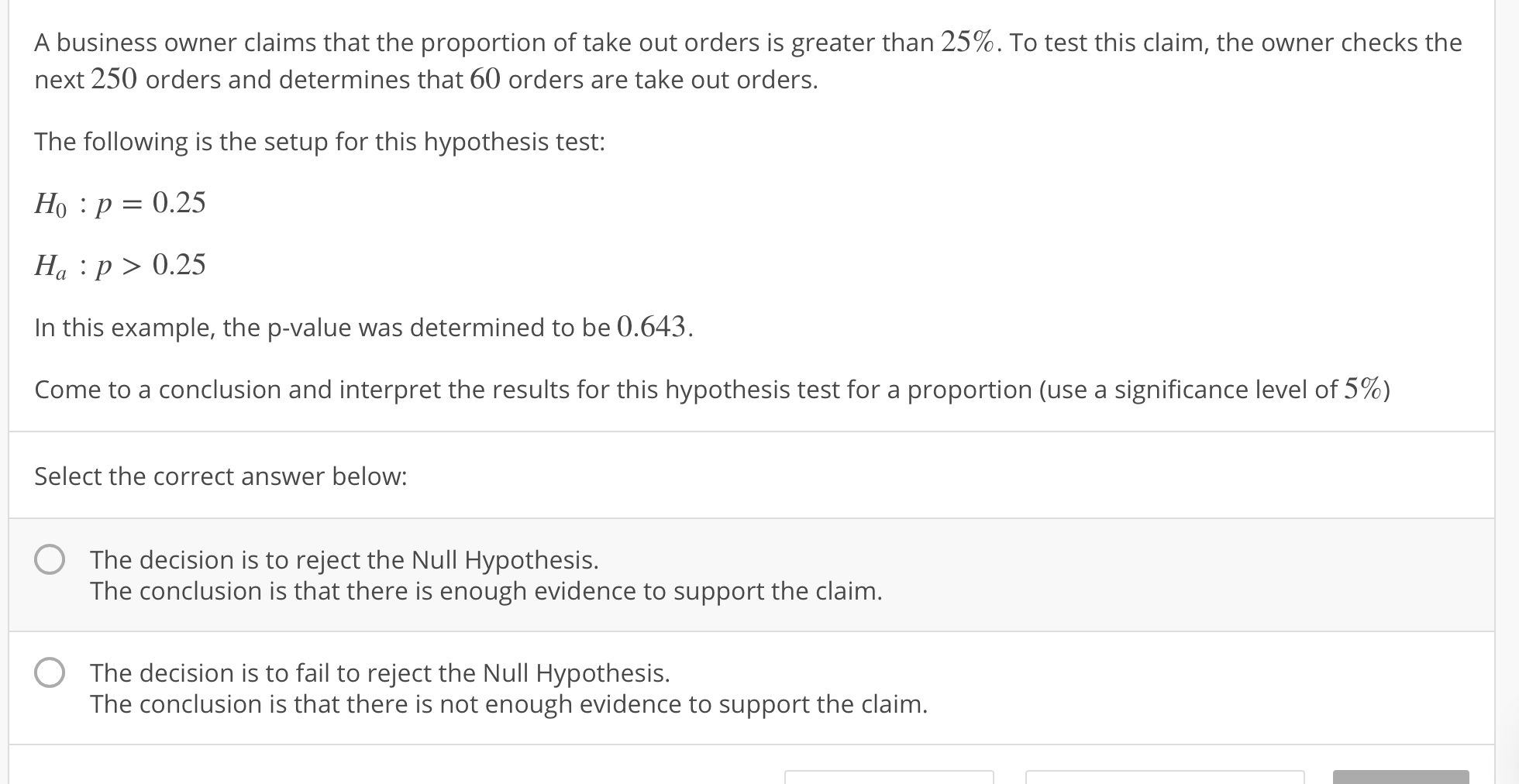# A business owner claims that the proportion of take out orders is greater than 25%. To test this claim, the owner checks thenext 250 orders and determines that 60 orders are take out ordersThe following is the setup for this hypothesis test:Ho :p 0.25Ha p>0.25In this example, the p-value was determined to be 0.643.come to a conclusion and interpret the results for this hypothesis test for a proportion (use a significance level of 5%)Select the correct answer below:OThe decision is to reject the Null Hypothesis.The conclusion is that there is enough evidence to support the claim.The decision is to fail to reject the Null Hypothesis.The conclusion is that there is not enough evidence to support the claim.

Question
486 viewshelp_outlineImage TranscriptioncloseA business owner claims that the proportion of take out orders is greater than 25%. To test this claim, the owner checks the next 250 orders and determines that 60 orders are take out orders The following is the setup for this hypothesis test: Ho :p 0.25 Ha p>0.25 In this example, the p-value was determined to be 0.643. come to a conclusion and interpret the results for this hypothesis test for a proportion (use a significance level of 5%) Select the correct answer below: O The decision is to reject the Null Hypothesis. The conclusion is that there is enough evidence to support the claim. The decision is to fail to reject the Null Hypothesis. The conclusion is that there is not enough evidence to support the claim. fullscreen
check_circle

Step 1

Let p be the proportion of proportion of take-out orders.

The aim is to check whether the proportion of take-out orders is greater than 25%.

The null and the alternative hypothesis is,

Step 2

Here the level of significance is 0.05 and the p–value is 0.643.

Decision based on the p–value method:

• If p ≤ α, then reject.

Conclusion:

Here α = 0.05, p– value = 0.643.

Therefore, the p– value is not less than the significance level.

That is, 0.643 > 0.05.

Using the decision rule, fail to reject the null hypothesi...

### Want to see the full answer?

See Solution

#### Want to see this answer and more?

Solutions are written by subject experts who are available 24/7. Questions are typically answered within 1 hour.*

See Solution
*Response times may vary by subject and question.
Tagged in

### Other